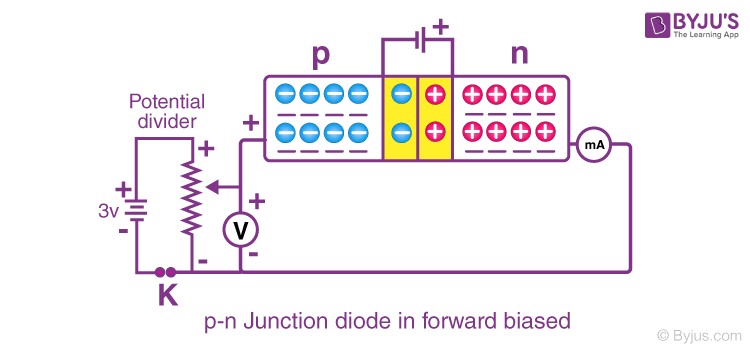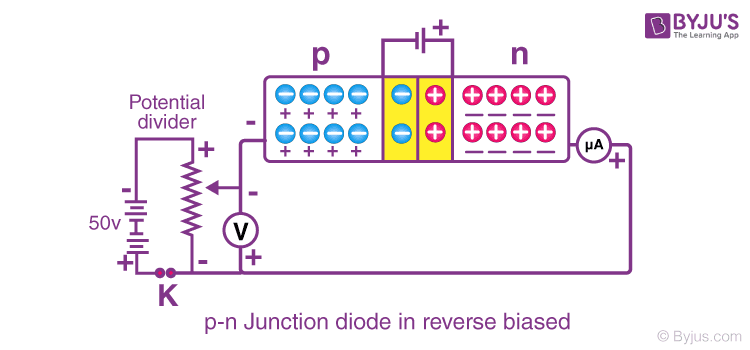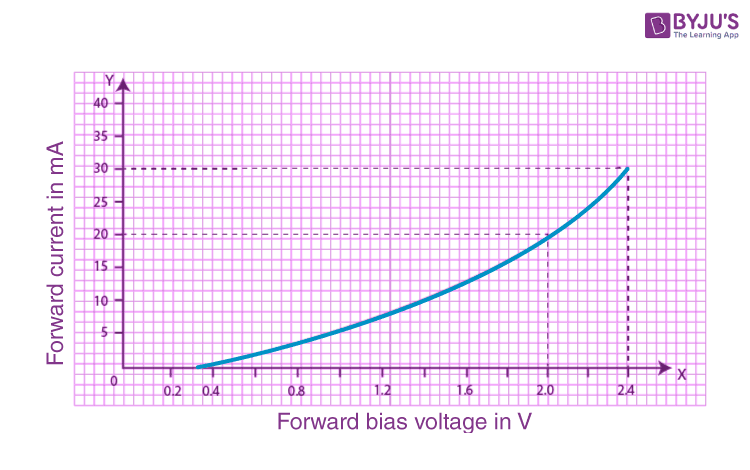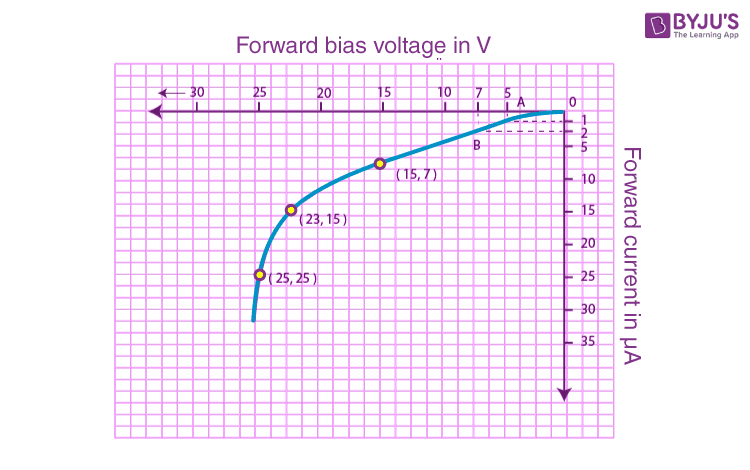# To draw the I-V characteristic curve for a p-n junction in forward bias and reverse bias

## Aim

To draw the I-V characteristic curve of a p-n junction in forward bias and reverse bias.

## Materials Required

1. A p-n junction diode
2. A 3-volt battery
3. A 50-volt battery
4. A high resistance rheostat
5. One 0-3 volt voltmeter
6. One 0-50 volt voltmeter
7. One 0-100 mA ammeter
8. One 0-100 μA ammeter
9. One way key
10. Connecting wires
11. Piece of sandpaper

## Theory

Forward bias characteristics

The junction is said to be forward biased when the p-section of the diode is connected to the positive terminal of the battery and the n-section of the diode is connected to the negative terminal of the battery. With an increase in the voltage, the current also increases. For Si diode, at 0.7 V the current increases suddenly.

Reverse bias characteristics

The junction is said to be reverse biased when the p-section of the diode is connected to the negative terminal of the battery and the n-section of the diode is connected to the positive terminal of the battery. With an increase in the voltage, there is a small change in the current but the reverse current increases to a higher value with an increase in the voltage.

## Diagram## Procedure

For forward-bias

1. The circuit connections should be as shown in the diagram.
2. All the connections should be neat, clean and tight.
3. For voltmeter (V) and milli-ammeter (mA), least count and zero error should be noted.
4. To get the zero reading from the voltmeter and milli-ammeter, rheostat should be brought near the negative end by inserting the key K.
5. To apply the forward bias voltage (VF) of 0.1V, the contact should be moved towards the positive end. The current remains zero.
6. Keeping current zero, increase the forward bias voltage up to 0.3 V for Ge diode.
7. To record a small current using milli-ammeter, increase the VF to 0.4 V.
8. Increase the VF by 0.2 V and record the corresponding current. When the VF becomes 0.7 V, the current will increase rapidly.
9. When VF = 0.72 V, the current increases suddenly and this is known as forward breakdown stage.
10. Take out the key if forward current won’t change as VF increased beyond forward breakdown.
11. Record the observations.

For reverse bias

1. The circuit connections should be as shown in the diagram.
2. All the connections should be neat, clean and tight.
3. Note the least count and zero error of voltmeter (V) and micro-ammeter (μA).
4. To get zero reading from the voltmeter V and micro-ammeter μA, insert the key K and bring the rheostat near the positive end.
5. To apply reverse bias voltage (VR) of 0.5 V, move the rheostat to the negative end so as to flow the reverse current.
6. Increase VR by 0.2 V and record the corresponding current. When VR becomes 20 V, the current will increase rapidly.
7. When VR = 25 V, the current increases suddenly and this is known as reverse breakdown stage. Record the current reading and take off the key.
8. Record the observations.

## Observations

For forward bias

Range of voltmeter = …….V

Least count of the voltmeter = …….V

Zero error of voltmeter = ……..V

Range of milli-ammeter = …….mA

Least count of milli-ammeter = …….mA

Zero error of milli-ammeter = ……..mA

Table for forward bias voltage and forward current

 Sl.no Forward bias voltage VF in V Forward current IF in mA

For reverse bias

Range of voltmeter = …….V

Least count of the voltmeter = …….V

Zero error of voltmeter = ……..V

Range of micro-ammeter = …….μA

Least count of micro-ammeter = …….μA

Zero error of micro-ammeter = ……..μA

Table for reverse bias voltage and reverse current

 Sl.no Reverse bias voltage VR in V Reverse current IR in μA

## Calculations

For forward bias

Plot a graph between VF and IF taking VF on the x-axis and IF on the y-axis. The graph obtained is known as forward bias characteristic curve.For reverse bias

Plot a graph between VR and IR taking VR on the x-axis and IR on the y-axis. The graph obtained is known as reverse bias characteristic curve.## Result

Junction resistance for forward bias = …….. ohms

Junction resistance for reverse bias = ……… ohms.

## Precautions

1. The connections should be neat, clean and tight.
2. Key should be used when the circuit is being used.
3. Beyond breakdown, forward bias voltage should not be applied.
4. Beyond breakdown, reverse bias voltage should not be applied.

## Sources Of Error

Faulty junction diode might be supplied.

## Viva Questions

Q1. Define energy level in an atom.

Ans: Energy level in an atom is defined as the energy value of an electron in the subshell of an atom.

Q2. What are the different types of energy bands?

Ans: Following are the different types of energy bands:

• Conduction band (C)
• Valence band (V)
• Forbidden band (F)

Q3. What are the different types of substances?

Ans: Following are the different types of substances:

• Conductors
• Insulators
• Semiconductors

Q4. What is the SI unit of conductance?

Ans: SI unit of conductance is siemens (S).

Q5. Name the different types of biasing.

Ans: Following are the different types of biasing:

• Forward biasing
• Reverse biasing